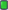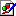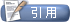MATLAB中国论坛|MATLAB爱好者之家—不仅仅是MATLAB![MATLAB工具箱] 新手请教优化的结果与边界约束值一样，请问是什么原因
 用户名 记住信息 密码
 注册账号 论坛帮助 会员列表 日历事件 搜索 今日新帖 标记版面已读2019-06-16, 12:47 PM   #1新手请教优化的结果与边界约束值一样，请问是什么原因

fmincon函数优化后 x（1）值正好是1200，与边界约束一样了

function f = gao_youhua(x)
f=2*1200*600*cos(20)/(1200+2*x(1))/sin(65);
end

function [c,ceq]= gao_con(x)
c(1)=-sin(20)*x(1)-600*sin(20);
c(2)=-x(2)+cos(20)*x(1)+650*cos(20);
c(3)=sqrt((600+x(1)*sin(60))^2+(x(2)-(600+x(1))*cos(60))^2)-sqrt((600+x(1)*sin(20))^2+(x(2)-(600+x(1))*cos(20)))*3;
ceq=[];
end遗传算法工具箱.JPG (80.4 KB, 0 次查看)fmincon函数.JPG (14.8 KB, 0 次查看)标签 优化 工具箱 结果 边界值主题工具显示可打印版本邮寄本页给好友 显示模式平板模式切换到混合模式切换到树形模式发帖规则 您不可以发表新主题 您可以发表回复 您不可以上传附件 您不可以编辑自己的帖子 启用 BB 代码 论坛启用 表情符号 论坛启用 [IMG] 代码 论坛禁用 HTML 代码Trackbacks are 禁用 Pingbacks are 禁用 Refbacks are 启用 版面规则

 -- 简体中文 -- 繁體中文 -- English (US) 联系我们 - MATLAB中国论坛|MATLAB爱好者之家 - 论坛存档 - 返回顶端